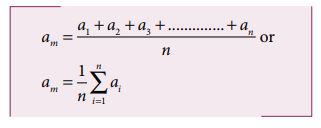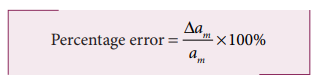Home | | Physics 11th std | Error Analysis

# Error Analysis

i. Absolute Error ii. Mean Absolute error iii. Relative error iv. Percentage error

Error Analysis

## i. Absolute Error

The difference between the true value and the measured value of a quantity is called absolute error. If a1, a2, a3, ………. an are the measured values of any quantity ‘a’ in an experiment performed n times, then the arithmetic mean of these values is called the true value (am) of the quantity.The absolute error in measured values is given by## ii. Mean Absolute error

The arithmetic mean of the magnitude of absolute errors in all the measurements is called the mean absolute error.If am is the true value and am is the mean absolute error then the magnitude of the quantity may lie between am + am and am -am

## iii. Relative error

The ratio of the mean absolute error to the mean value is called relative error. This is also called as fractional error (or) relative error.Thus Relative error expresses how large the absolute error is compared to the total size of the object measured. For example, a driver’s speedometer shows that his car is travelling at 60 km h-1 when it is actually moving at 62 kmh-1. Then absolute error of speedometer is 62-60 km h-1 = 2 km h-1 Relative error of the measurement is 2 km h-1 / 60 km h-1 = 0.033.

## iv. Percentage error

The relative error expressed as a percentage is called percentage error.A percentage error very close to zero means one is close to the targeted value, which is good and acceptable. It is always necessary to understand whether error is due to impression of equipment used or a mistake in the experimentation.

Example 1.4

In a series of successive measurements in an experiment, the readings of the period of oscillation of a simple pendulum were found to be 2.63s, 2.56 s, 2.42s, 2.71s and 2.80s. Calculate (i) the mean value of the period of oscillation (ii) the absolute error in each measurement (iii) the mean absolute error (iv) the relative error (v) the percentage error. Express the result in proper form.

SolutionTags : Absolute, Mean Absolute, Relative, Percentage error | Theory of Errors | Physics , 11th Physics : UNIT 1 : Nature of Physical World and Measurement
Study Material, Lecturing Notes, Assignment, Reference, Wiki description explanation, brief detail
11th Physics : UNIT 1 : Nature of Physical World and Measurement : Error Analysis | Absolute, Mean Absolute, Relative, Percentage error | Theory of Errors | Physics